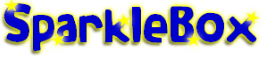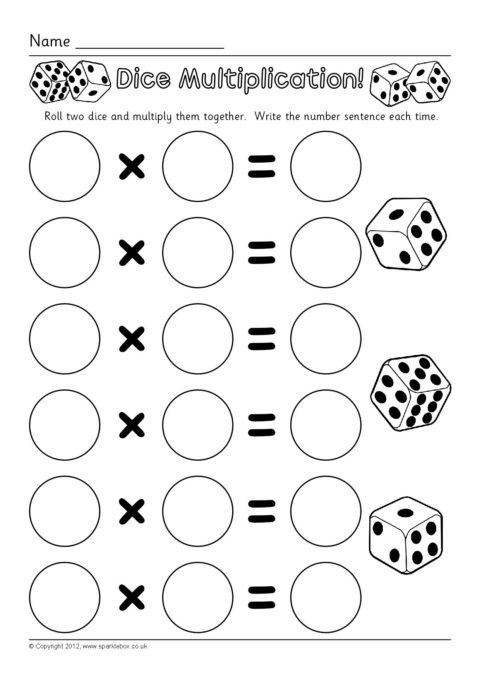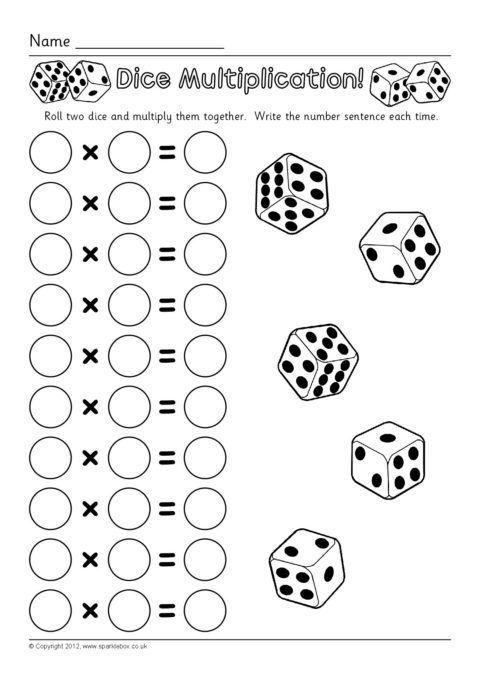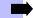Find ResourcesDice Multiplication Worksheets

Dice Multiplication Worksheets

Description

Simple worksheets where pupils roll two dice, multiply the numbers together and write the number sentence.

Simple worksheets where pupils roll two dice, multiply the numbers together and write the number sentence.

PreviewRelated ItemsMultiplication ResourcesTimes Table Resources